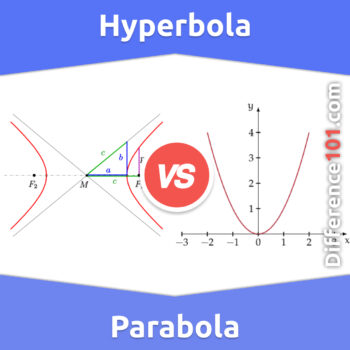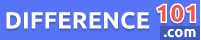# Hyperbola vs. Parabola: What Is The Difference Between Hyperbola And Parabola?

Hyperbolas and parabolas are two types of conic sections that have important mathematical and physical applications. While they share some similarities, they have distinct differences. Read more here.When it comes to conic sections, the hyperbola and parabola are two of the most common curves that we come across. They are both essential in mathematics, physics, engineering, and other fields. However, despite their similarities, they have distinct characteristics that set them apart. Understanding the difference between the hyperbola and parabola is crucial for anyone dealing with these curves.

## What Is Hyperbola?A hyperbola is a mathematical curve that is formed when a plane slices a double cone almost parallel to its axis. The resulting shape of the curve is symmetrical, with two separate branches that are identical in shape but facing different directions. The hyperbola is defined by two focal points, which are equidistant from the center of the curve, and the two axes of the curve – the transverse axis and the conjugate axis. The hyperbola can be found in many applications, including astronomy, physics, and engineering. Its properties and characteristics have been studied extensively by mathematicians over the centuries, making it an important part of the mathematics curriculum.

## What Is Parabola?A parabola is a mathematical curve that is formed when a plane intersects with a cone at an angle that is parallel to one of its sides. This shape is defined by a quadratic equation, which makes it a conic section, and it represents an important aspect of geometry and physics. Parabolas have a wide range of applications, including in rocketry and optics, and they are often used to model the trajectory of objects in motion. Beams of light and sound waves can also be focused using parabolic reflectors, which makes this curve a crucial element in modern technology. Overall, understanding the properties of the parabola is essential for anyone interested in the fields of mathematics, engineering, or physics.

## Key Differences Between Hyperbola And Parabola

Hyperbola and Parabola are two of the most commonly used curves in mathematics. The key difference between these curves lies in the orientation of the plane with respect to the cone axis. A Hyperbola is formed when the plane cuts almost parallel to the axis, resulting in two U-shaped curves that are mirror images of each other. On the other hand, a Parabola is formed when the plane cuts parallel to the cone side, resulting in a downward opening curve that has a focus point and directrix. Moreover, Hyperbolas have two foci and two vertices, whereas Parabolas have one focus and one vertex. In summary, the orientation of the plane is the main difference between Hyperbola and Parabola.

1. A hyperbola is obtained by the plane cutting almost parallel to the axis.
2. A parabola is obtained by the plane cutting parallel to the cone side.
3. Hyperbolas have two foci and two vertices, whereas Parabolas have one focus and one vertex.
👉  Quad cab vs. Crew cab: What is the difference between Quad cab and Crew cab?

## Hyperbola vs. Parabola Similarities

Hyperbola and Parabola are two types of conic sections popularly used in mathematics. Although both curves possess different characteristics, they also share a few similarities. One such similarity is that both curves have an axis of symmetry. Another similarity between the two is their focus and directrix properties. Both Hyperbola and Parabola have a focus point that lies along the axis of symmetry and a directrix that is perpendicular to it. Additionally, as they are obtained by cutting a cone, both curves have a common vertex. Although there are slight differences between the two curves, these similarities prove useful in understanding their properties and applications in various areas of mathematics, physics and engineering.

1. One such similarity is that both curves have an axis of symmetry.
2. Both Hyperbola and Parabola have a focus point that lies along the axis of symmetry and a directrix that is perpendicular to it.
3. The graph of a hyperbola is concave upward.
4. As they are obtained by cutting a cone, both curves have a common vertex.

## Hyperbola vs. Parabola Pros and Cons

### Hyperbola Pros & Cons#### Hyperbola Pros

Hyperbola is a popular curve in mathematics, which is obtained when a plane cuts almost parallel to the axis. This curve has several advantages and pros, making it an important tool for various applications. Firstly, hyperbolas are used in optics, specifically in the construction of optical hyperboloids that are used in telescopes and satellite dishes. It also has applications in astrophysics, where it helps to understand the movement of celestial bodies. The curve’s properties are also used in engineering to design curved surfaces, such as those found in the hulls of ships and airplane wings. Lastly, the hyperbola is an essential concept in graph theory, where it is used to represent networks and social systems. The advantages of this curve make it an invaluable tool in various fields of study.

1. Hyperbolas are used in optics, specifically in the construction of optical hyperboloids that are used in telescopes and satellite dishes.
2. It also has applications in astrophysics, where it helps to understand the movement of celestial bodies.
3. The hyperbola is an essential concept in graph theory, where it is used to represent networks and social systems.
👉  Genus vs. Species: Difference Between Genus and Species

#### Hyperbola Cons

The Hyperbola, although an important geometrical figure, has several disadvantages and cons that must be duly noted. Firstly, it is a difficult curve to work with as it can be quite complex and requires advanced mathematical skills to understand and manipulate. Additionally, its asymmetrical nature makes it challenging to apply in practical applications with symmetry. Furthermore, in certain cases, hyperbolas can produce multiple answers, leading to confusion and multiple interpretations. Lastly, it is not as commonly used in everyday life, which further reduces its practicality. Therefore, while the hyperbola is critical, its disadvantages and cons must be duly noted for proper understanding and utilization.

1. It is a difficult curve to work with as it can be quite complex and requires advanced mathematical skills to understand and manipulate.
2. Its asymmetrical nature makes it challenging to apply in practical applications with symmetry.
3. In certain cases, hyperbolas can produce multiple answers, leading to confusion and multiple interpretations.

### Parabola Pros & Cons#### Parabola Pros

The parabola is a mathematical curve that is formed when a plane intersects parallel to a cone’s side. The Parabola has several advantages for various applications. Firstly, it is widely used in physics to illustrate the trajectory of a projectile. This property makes the parabola an essential tool in designing bridges, buildings, and even telescopes. Secondly, parabolic reflectors have become popular in architecture, lighting design, and satellite dish technology. Finally, the parabolic curve is symmetrical and can be used to reflect light or sound waves equally. This feature makes it ideal for creating high-gain directional antennas and loudspeakers. In summary, the parabola offers several significant benefits in various fields, making it an essential mathematical tool.

1. It is widely used in physics to illustrate the trajectory of a projectile.
2. Parabolic reflectors have become popular in architecture, lighting design, and satellite dish technology.
3. The parabolic curve is symmetrical and can be used to reflect light or sound waves equally.
👉  Graphic Card vs. Video Card: What Is the Difference Between Graphic Card and Video Card?

#### Parabola Cons

Parabolas are a commonly studied curve in mathematics, however, they are not without their disadvantages and cons. One of the most significant drawbacks of a parabola is its limited range. The curve continues indefinitely in both directions but eventually flattens out to a horizontal line, which means that it has limited practical applications. Also, parabolas have limited symmetry, notably only one line of symmetry, limiting their potential use in applications that require symmetrical shapes. Moreover, the nature of the curve makes it difficult to approximate or estimate surface area and length in three dimensions. In addition, parabolic shapes can be challenging to manufacture or machine, limiting their practical applications.

1. One of the most significant drawbacks of a parabola is its limited range.
2. The curve continues indefinitely in both directions but eventually flattens out to a horizontal line, which means that it has limited practical applications.
3. Parabolas have limited symmetry, notably only one line of symmetry, limiting their potential use in applications that require symmetrical shapes.

## Comparison Chart## Conclusion

In conclusion, hyperbolas and parabolas are both important mathematical concepts with unique characteristics. While hyperbolas are obtained when a plane cuts almost parallel to the axis of a cone, parabolas are obtained when the plane cuts parallel to the cone side. The shape and properties of these curves make them valuable in many fields, including physics, engineering, and architecture. Understanding the differences between hyperbolas and parabolas is crucial for solving complex mathematical problems and creating practical solutions in the real world.Michael Dorns

Michael Dorns is a media researcher and investigator at Difference 101. He graduated from California State University, Los Angeles, with a B.A. in English literature. He enjoys American literature, technology, animals, and sports. Michael has lived in four different countries on three continents and has also visited forty-two states and thirty-three countries. He currently resides in Los Angeles, California, with his wife and two children.

We will be happy to hear your thoughts